Next: Conclusion Up: Experiments and results Previous: Description

## Results

First, projective displacements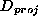are calculated from point correspondences and epipolar geometry with the method described in [HC98]. Then, the equation of the plane at infinity is calculated and the infinite homographies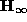associated to the left camera are derived. Our affine calibration algorithms are similar to [BZ95] and [HC98] and cope with general and planar motions (in this case, we need at least 2 motions). Finally, the real Jordan decomposition of eachis calculated and the resolution of eq:kkt enables us to obtain the complete camera calibration.

We show the results on 4 motion sequences (each consisting of 5 motions) :

sequence 1 : non singular general motions sequence 2 : non singular planar motions sequence 3 : planar motion with a horizontal rotation axis sequence 4 : planar motion with a vertical rotation axis

The following table exhibits the matricesand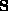obtained for the first motion of each sequence. It confirms the particular forms ofobtained for critical motions.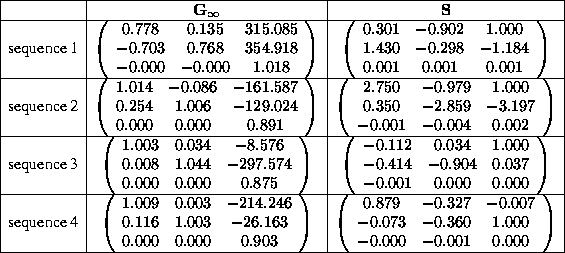The results of the computation of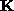from the previous matricesare as follows :

with the assumption r=0, only the first two sequences allow us to calculate: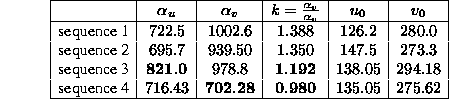the assumption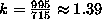allows us to calculatein all sequences :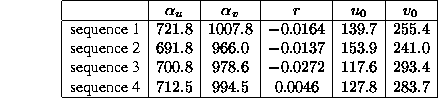From these experiments, we can see clearly that the k-constraint allows us to calibrate even in the case of critical motions. With the r-constraint, we can see that significant errors are made in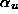for sequence 3, and in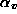for sequence 4.

Bob Fisher
Mon Dec 7 13:48:06 GMT 1998